# Lucky Dice

#### Panda

Staff member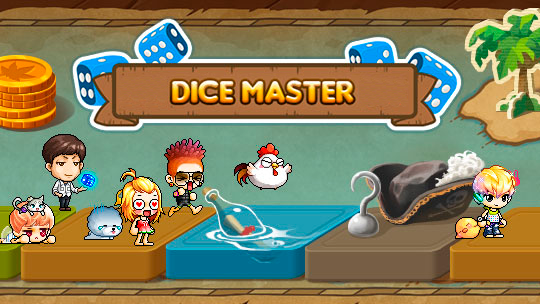It is a mini-game where you can bet your Donation Point on the winning die with the highest score and double your bet.How does it work?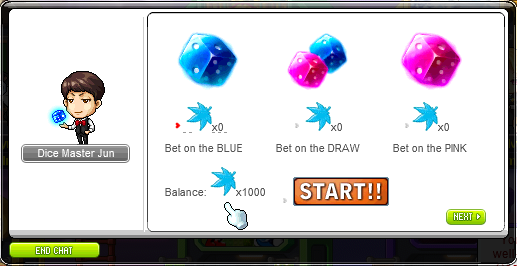•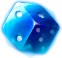= Multiply the amount wagered x2.
•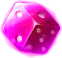= Multiply the amount wagered x2.
•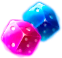= Multiplies the amount bet according to the sum of the dice.
Example:
If the BLUE die rolls 2 and the PINK die rolls 2, the total sum is 4, and the bet will be multiplied by 4.How can I play?

In order to enter the Mini-Game we must talk to the NPC Dice Master Jun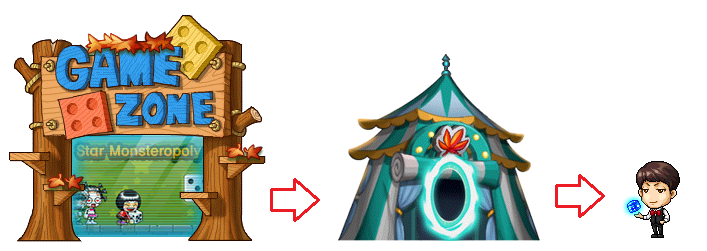In this window we will choose the die we want to bet and then we will choose the amount of Donation Point to bet.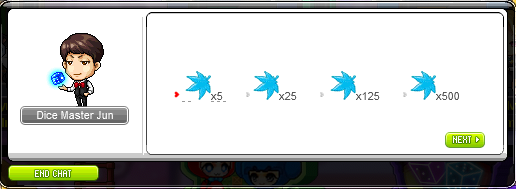NOTE :
• If we choose the middle dice, it means that we will bet on a tie (i.e. if the blue and pink dice have the same number).
• We can also make multiple bets (choose the other die or tie).Example N°1 : We will choose the Blue die, bet x25 Donation Point and then click on START!!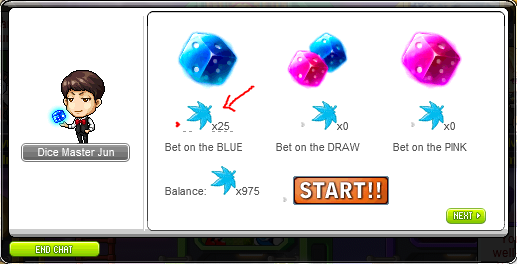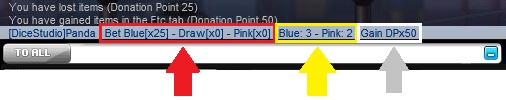• RED Arrow : This indicates the amount wagered on the die or tie option (BLUE = blue die | DRAW = tie | PINK = pink die).
• YELLOW ARROW : This indicates the amount rolled on each die.
• GREY ARROW : This indicates the amount of Donation Point earned.Example N°2 : We will bet x25 Donation Point on the BLUE die and x5 Donation Point on the PINK die.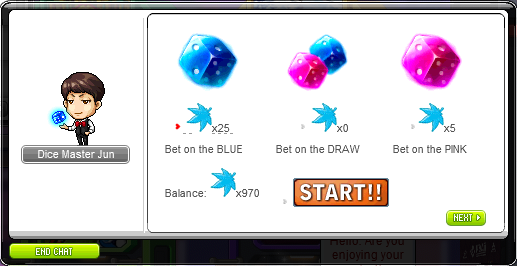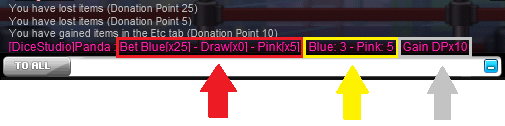• RED Arrow : Here we bet x25 on BLUE and x5 on PINK.
• YELLOW arrow : Here it indicates that the BLUE die rolled 3 and the PINK die rolled 5 (the PINK die won because it was the highest number).
• GRAY Arrow : Here we gain x10 Donation Point (PINK die multiplies it x2).Example N°3 : We will bet x125 Donation Point on the tie (middle dice option).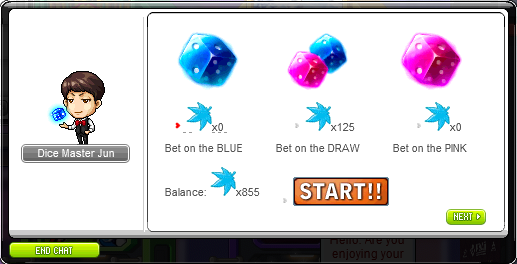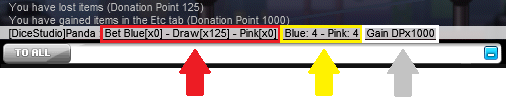• RED ARROW : This indicates that we bet x125 on the tie.
• YELLOW Arrow : This indicates that both dice rolled 4.
• GREY Arrow : Here we earn x1000 Donation Point (since the sum of the dice is 8, then it would be 125DP x 8 = 1000DP).

 ​NOTE :​ Remember to always check that you have space in your inventory before using the Mini-Game. When a wager is entered in any of the 3 options, the wager cannot be removed and the Donation Points will remain stored there and will be discounted from your inventory.

Last edited: